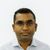# App Development

Announcements
Qlik Cloud Maintenance is scheduled between March 27-30. Visit Qlik Cloud Status page for more details.
cancel
Showing results for
Did you mean:Contributor III

## how to count data

Hi,

I am creating the sample data table  here :

 Code Category Key 112 A 001236 112 A 002589 112 A 002574 456 B 003698 456 B 007896 890 A 002365 890 A 005987 890 A 002365 342 B 001478

.i have 3 fields i want count of "key" of all code where "category" = A. I tried aggr function in expression but did not to get the expected answer.

my input is Code. for example: when I select code (112 ) it belongs to category A . so my expected answer is count of all key which has category A.

Expected result:      A =  count key = " 6 "

i have tried this expression but it gives me different answer.

Sum(Aggr(Count(Key),category)

Thanks!

Labels (1)
• ### General Question

1 Solution

Accepted SolutionsMVP

Can u try this?

``=Count({1<Category=p()>} Key)``
8 RepliesMVP

Try this,``=Count({1<Category=p()>} TOTAL Key)``Contributor III
Author

Thanks Saravanan , yes your output is correct but it didn't work for me it gave me total count key of all categories. I really appreciated.MVP

Can u try this?

``=Count({1<Category=p()>} Key)``Contributor III
Author

no, it does not work either. Thanks!MVP

Can you describe how you tried to implement the proposed solution and how your results are different from your expectation?Contributor III
Author

it worked perfectly with this sample inline table :

=Count({1<Category=p()>} Key)

Test:
Code,Category,Key
1122, A, 6
1122, A,8
1122, A, 5
456, B, 7
456, B, 1
890, A, 2
890, A, 3
789, B, 4
789, B, 9
678, C, 10
678, C, 12
]
;

Result:

A= 5

B= 4

C=2

i have huge data of 10 categories and hundreds of code , the above expression should work for  it too but dont know why it does not work.Contributor III
Author

Thanks Saravanan , it works actually i didnt put filters in set analysis. now values are correct. I really appreciated, Thanks again one more time.Contributor III
Author

Hello , I need to use above logic with AGGR() function . below is the logic :

below logic when I selected Customer Code (which belongs to finance dept) I get from below logic DATE - DATE / SUM of that code . but I need to get all codes of same dept when I selected Customer Code .

/////////////////////////////////////////////////////////////////////////////////////

ceil((sum(aggr(distinct min({<[Cat Type]= {'102'}, finalCheck ={"1"},Weeks-={"0"}> } [ Date])

-

(if([VFlag] = '1' AND [PN1] = [PN2],

If(PO=[PO 2] and Not Isnull([BN 2]),

aggr(min({<[Cat Type] , finalCheck ={"1"},Weeks -= {"0"}>} [Date]),key)),

if( not isnull (min([Release Date])),
aggr(min({<[Cat Type]= {'102'} , finalCheck ={"1"}, Weeks-= {"0"}>} [Release Date]),key),

[Creation Date]))),key)))/7)

/

Sum(aggr({<finalCheck={"1"}, [Cat Type]= {'102'} >} finalCheck ,key))

////////////////////////////////////////////////////////////////////////////////

the above logic works perfect for when I selected Customer code I will get the sum of days . I need when I selected the Customer code I will get all customer codes which belong to same dept .Tags
Community Browser